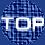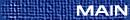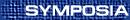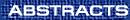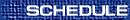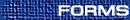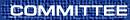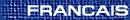Infinite Dimensional Lie Theory and Representation Theory / Théorie de Lie en dimension infinie et théorie des représentations
(Stephen Berman, Organizer)

BRUCE ALLISON, University of Alberta, Edmonton, Alberta
Extended affine Lie algebras of nonreduced type

Extended affine Lie algebras are higher nullity generalizations of affine Kac-Moody Lie algebras. The structure of extended affine Lie algebras when the associated finite root system has reduced type is now fairly well understood. In this talk we report on recent progress in the case when the root system has nonreduced type.

Classical Lie (super)algebras over associative algebras

>From matrices over simple associative domains and their one-sided ideals, we construct families of simple Lie algebras and Lie superalgebras. Our construction intermixes the structure of the associative algebra and the structure of the matrix algebra. The resulting Lie (super)algebras have a grading by a finite root system. This is joint work with X. Xu and K. Zhao.

YULY BILLIG, Carleton
To be announced

DAN BRITTEN, University of Windsor, Windsor, Ontario
On the lattice of submodules of generalized Verma modules

Let G be a finite dimensional simple Lie algebra over the complex numbers with semi-simple regular subalgebra [^(G)]. Let F be a torsion free [^(G)]-module and P be the parabolic subalgebra of G generated by [^(G)], the Cartan subalgebra of G and all positive root vectors of G. Fix a basis of h1,..., hl of annH([^(G)])={h Î H | [h,[^(G)]]=0} and define a P-module structure on F by

 Xa·F=0    for all positive root vectorsXa Ï ^G
 hi·F=ai   with ai arbitrary complex numbers.
Our aim is to study the lattice of submodules of the generalized Verma module, GVM, over U(G) define by parabolic induction,

 M(F:a1,...,al)=U(G) Å U(P) F.
In particular, the lattice of submodules of M(F:a1,...,al) is related to the lattice of submodules within a quotient module of a Verma modulo a sum of its Verma submodules. Through this relationship an analogue of the BGG criterion is obtained for GVMs.

(Joint work with V. M. Futorny and F. W. Lemire)

CHONGYING DONG, California, Santa Cruz
To be announced

TERRY GANNON, Alberta
To be announced

YUN GAO, York
Double affine algebras

MARIA GOLENISHCHEVA-KUTUZOVA, University of Florida, Department of Mathematics, Gainesville, Florida  32611-8105, USA
G-conformal algebras

G-conformal algebra is an axiomatic description of the operator product expansion of chiral fields with simple poles at finitely many points. We classify these algebras and their representations interms of Lie algebras and their representations with an action of the group G. To every G-conformal algebra and a character of G we associate a Lie algebra generated by fields with the OPE with simple poles. Examples include twisted affine Kac-Moody algebras, the sin-algebra (which is a G-conformal'' analogue of the general linear algebra) and the algebra of pseudodifferential operators on the circle.

BOB GRIESS, Ann Arbor
To be announced

FRANK LEMIRE, University of Windsor, Windsor, Ontario
A duality theorem for torsion free modules of symplectic Lie algebras

Let L denote a semisimple complex Lie algebra with Cartan subalgebra H and let P denote a parabolic subalgebra of L with P=[(L)\tilde]Å[(H)\tilde]ÅN ([(L)\tilde] is a regular semisimple subalgebra of L, [(H)\tilde] is the complement of the Cartan subalgebra of [(L)\tilde] orthogonal to [(L)\tilde] in H and N is the nilpotent part of P). Let L denote the category of all torsion free [(L)\tilde] modules of finite degree. Following [FKM] we define O(P,L) to be the category of all finitely generated, N finite L modules which when viewed as [(L)\tilde] modules decompose into a direct sum of modules in L and the kernels of all L module homomorphisms when viewed as [(L)\tilde] modules decompose into a direct sum of modules in L. When [(L)\tilde] is trivial this category reduces to the classical category O.

In category O the classical BGG reciprocity theory states that there is a bijective correspondence between indecomposable projective modules and simple modules and further that, using Verma modules as intermediaries, here is a reciprocity between the multiplicity of a Verma module in an indecomposable projective module and the multiplicity of the corresponding simple module in the Verma module. In order to provide an analogous reciprocity theorem for the category O(P,L) Futorny et. al. assumed a so called duality property-i.e. for any two simple modules V, W Î L there exists a constant i(V,W) such that for any finite dimensional [(L)\tilde] module F we have [FÄV:W]=i(V,W)[FÄW:V]. They also establish that if [(L)\tilde] @ sl (2,C) the assumption is valid.

In this talk we show that this duality property holds whenever [(L)\tilde] @ sp (2n,C) with i(V,W)=1 for all simple modules V,W however the duality property is not valid when [(L)\tilde] @ sl (n,C) for n ³ 3.

References

[FKM ] V. Futorny, S. Konig and V. Mazorchuk, Categories of Induced Modules and Projectively Stratified Algebra. 1999, preprint.

HAI SHENG LI, Rutgers, The State University of New Jersey, Camden, New Jersey  08102, USA
A certain higher dimensional analogue of vertex algebras

A higher dimensional analogue of the notion of vertex algebra is formulated in terms of formal variable language with Borcherds' notion of G-vertex algebra as a motivation. Some examples are given and certain analogous duality properties are proved. Furthermore, it is proved that for any vector space W, any set of mutually local multi-variable vertex operators on W generates in a certain canonical way a vertex algebra with W as a natural module.

KIYOKAZU NAGATOMO, Osaka University, Department of Mathematic, Graduate School of Science, Osaka University, Toyonaka, Osaka  560-0043, Japan
Finiteness of conformal blocks and C2 finiteness

We first give the definition of the conformal blocks over compact Riemann surfaces based on vertex operator algebras. Then we will show that if a vertex operator algebra V and their irreducible modules satisfy C2 finiteness condition then the corresponding conformal blocks are all finite dimensional. In particular this shows the finiteness of the conforma blocks for both WZNW model and the minimal model, which correspond to affine Lie algebras and the Virasoro algebra, respectively.

ERHART NEHER, University of Ottawa, Ottawa, Ontario  K1N 6N5

Lie superalgebras graded by root systems are Lie superalgebras L with a grading L=Åa Î RÈ{0} La, [La,Lb] Ì La+ b for a,b Î RÈ{0}, which is induced in a suitable sense by a split simple subalgebra G of L[`0], the even part of L. Here R is the root system of G, and this may be finite or only locally finite, in which case G is finite-dimensional respectively locally-finite.

The corresponding Lie algebras have recently been investigated by several authors, among them Allison, Benkart, Berman, Gao, Moody, Zelmanov and the speaker. One reason for studying these Lie algebras is that they enter in the construction of extended affine Lie algebras. In the case of infinite root systems special examples turn up in the representation theory of affine Lie algebras. The superversions of these algebras have recently been investigated by Cheng and Wang.

In the talk I will describe my recent joint work with Esther Garcia (Oviedo) in which we describe Lie superalgebras graded by 3-graded root systems, i.e., R is not of type E8, F4 or G2. In this case, the Lie superalgebras are central extensions of the Tits-Kantor-Koecher superalgebra of a certain class of Jordan superpairs which I have recently classified. An important ingredient in the description of these Lie superalgebras is their supercoordinate system. It can for example be used to describe simplicity or the Gelfand-Kirillov dimension.

ARTURO PIANZOLA, University of Alberta, Edmonton, Alberta  T6G 2G1
A geometric interpretation of affine Kac-Moody Lie algebra

We will review Kac's realization of the affine algebras as twisted loop algebras. The main idea is then to interpret twisted loop algebras in general, as principal homogeneous spaces over the ring of Laurent polynomials.

YOJI YOSHII, University of Alberta, Edmonton Alberta  T6G 2G1
A simple characterization of the core of an EALA

It is important to consider affine Lie algebras as Z-graded algebras. In this spirit we consider the core of an extended affine Lie algebra (EALA) as a Zn-graded algebra. We call a certain Zn-graded Lie algebra a Lie n-torus or simply a Lie torus. A Lie torus is a natural generalization of the core of an EALA. It turns out that there exists a nontrivial graded form on any Lie torus, and this gives us a simple characterization of the core of an EALA. Namely, the core of any EALA is a Lie torus and any Lie torus is centrally isogeneous to some core of an EALA. For example, a Lie 1-torus is exactly a (twisted) loop algebra or its universal covering.

MIKHAIL ZAICEV, Moscow
To be announced